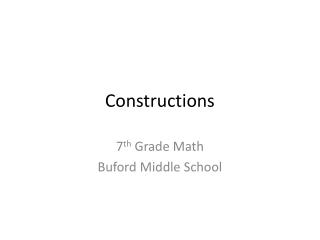DownloadDownload PresentationConstructions

# Constructions

Download Presentation## Constructions

- - - - - - - - - - - - - - - - - - - - - - - - - - - E N D - - - - - - - - - - - - - - - - - - - - - - - - - - -
##### Presentation Transcript

1. Constructions 7th Grade Math Buford Middle School

2. What are constructions? • Constructions are geometric “pictures” that are created using a straightedge and a compass.

3. Construction Vocabulary • Line Segment – a part of a line that has two endpoints. The two endpoints are used to name the line. • Ray – a part of a line with one endpoint. Rays are named using its endpoint first and then any other point on the ray. • Angle – a figure formed by two rays that have the same endpoints. That endpoint is called the vertex. You name and angle using 3 points: a point on one ray, the vertex, and a point on the other ray

4. Which figure has exactly two endpoints? • Angle • Line • Line segment • ray

5. Which statement describes angle QNT? • Its vertex is point N. • Its vertex is point Q. • Its vertex is point T. • It has no vertex.

6. To bisect an angle means to ____. • Copy it • Cut it in half • Double its size • Rotate it

7. Joel wants to bisect angle FGH. What should he do first? • Swing an arc from point F • Swing an arc from point G. • Swing an arc from point H • Use his straightedge to draw a segment.

8. How is the figure shown below named? • Ray UT • Line UT • Ray TU • Line TU

9. Which of the following can NOT be used to name the angle shown below? • Angle BAT • Angle CAT • Angle TAB • Angle TBC

10. If two lines lie in the same plane and do NOT intersect, they are called ____. • Bisectors • Horizontal • Parallel • Perpendicular

11. Uri wants to draw a line through point P that is parallel to line segment KD. What is the first step Uri should take. • Use a straightedge to draw a segment through point P that crosses line KD. • Use a straightedge to draw a segment through points K and D. • Swing an arc from point P. • Swing equal arc from point P. • Swing equal arcs from points K and D.

12. A line segment has length of 36 cm. If Lu bisects the segment, what is the length of each segment he constructs? • 12 cm • 18 cm • 36 cm • 72 cm

13. Janna wants to draw a line through point D that is perpendicular to line segment QV. What is the first step Janna should take? • Use a straightedge to draw a segment through point D. • Use a straightedge to draw a segment through points Q and V. • Swing and arc from point D. • Swing equal arcs from points Q and V.

14. To bisect line PQ, Zena began by swinging equal arcs from points P and Q above the segment and labeling point X where the arcs met. What should Zena do next? • Use a straightedge to draw a line segment from X to P and a line segment from X to Q. • Use a straightedge to draw a line segment from X to the point in the middle of line PQ. • Swing an arc from point X. • Swing equal arcs from points P and Q below the segment.